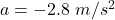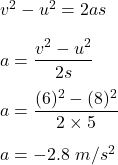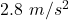## A speed skater moving to the left across frictionless ice at 8.0 m/s hits a 5.0-m-wide patch of rough ice. She slows steadily, then continue

Question

A speed skater moving to the left across frictionless ice at 8.0 m/s hits a 5.0-m-wide patch of rough ice. She slows steadily, then continues on at 6.0 m/s. What is her acceleration on the rough ice?

in progress 0
2 months 2021-07-29T10:40:33+00:00 1 Answers 9 views 0

Acceleration,Explanation:

It is given that,

The initial speed of a speed skater, u = 8 m/s

The final speed of a speed skater, v = 6 m/s

Width of patch of rough ice, s = 5 m

We need to find the acceleration on the rough ice. Acceleration can be calculated using third equation of motion as :So, the acceleration on the rough ice is. Negative sign shows that its speed is decreased.